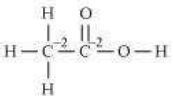8.2 What are the oxidation number of the underlined elements in each of the following and how do you rationalise your results ?

(a) KI3 (b) H2S4O6 (c) Fe3O4 (d) CH3CH2OH (e) CH3COOH

(a) KI${}_{3}$
In KI${}_{3}$, the oxidation number (O.N.) of K is +1. Hence, the average oxidation number of I is $\frac{1}{3}$. However, O.N. cannot be fractional. Therefore, we will have to consider the structure of KI${}_{3}$ to find the oxidation states. In a KI${}_{3}$ molecule, an atom of iodine forms a coordinate covalent bond with an iodine

molecule.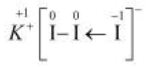Hence, in a KI3 molecule, the O.N. of the two I atoms forming the I2 molecule is 0, whereas the O.N. of the I atom forming the coordinate bond is –1.

(b) H${}_{2}$S${}_{4}$O${}_{6}$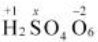Now, 2(+1)+4(x)+6(-2)=0

$⇒$2+4x-12=0

$⇒$4x=10

$⇒$x=+2$\frac{1}{2}$

However, O.N. cannot be fractional. Hence, S must be present in different oxidation states in the molecule.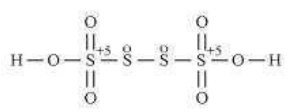The O.N. of two of the four S atoms is +5 and the O.N. of the other two S atoms is 0.

(c) $\overline{)\mathrm{F}}{\mathrm{e}}_{3}{\mathrm{O}}_{4}$

On taking the O.N. of O as –2, the O.N. of Fe is found to be $+2\frac{2}{3}$. However, O.N. cannot be fractional.
Here, one of the three Fe atoms exhibits the O.N. of +2 and the other two Fe atoms exhibit the O.N. of +3.

$\stackrel{+2}{\mathrm{F}}\mathrm{eO},\stackrel{+3}{\mathrm{F}}{\mathrm{e}}_{2}{\mathrm{O}}_{3}$

(d)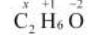2(x)+4(+1)+1(-2)=0

$⇒$ 2x+6-2=0

$⇒$x=-2

Hence, the O.N. of C is –2.

(e) ${\mathrm{CH}}_{3}\overline{)C}\mathrm{OOH}$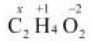2(x)+4(+1)+2(-2)=0

$⇒$2x+4-4=0

$⇒$x=0

However, 0 is average O.N. of C. The two carbon atoms present in this molecule are present in different environments. Hence, they cannot have the same oxidation number.
Thus, C exhibits the oxidation states of +2 and –2 in CH3COOH.